# Equal Shares - Definition with Examples

The Complete K-5 Math Learning Program Built for Your Child

• 40 Million Kids

Loved by kids and parent worldwide

• 50,000 Schools

Trusted by teachers across schools

• Comprehensive Curriculum

Aligned to Common Core

What is Equal Shares?

On dividing the whole or a group of objects is into equal parts, we get equal shares. We need to divide an object or a whole number into equal parts to distribute it equally. These equal parts have to be the same in measurements like weight, volume, dimensions, numbers etc.

Equal shares of a group of objects:

When shares are equal, each share or group has the same number of objects. Equal shares can be done in many ways depending upon the number of shares or portions we want to create.

For example, 10 kites can be equally grouped as follows:There are 10 kites.

There are 5 groups.

Each group has 2 kites.

5 groups with 2 kites = 10 kitesThere are 10 kites.

There are 2 groups.

Each group has 5 kites.

2 groups with 5 kites  = 10 kites

Examples and non-examples of equal shares of a group of objects:

 Equal Shares Unequal Shares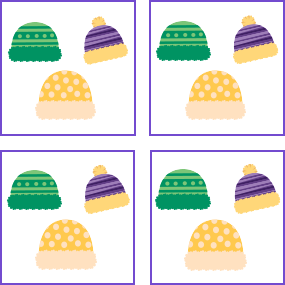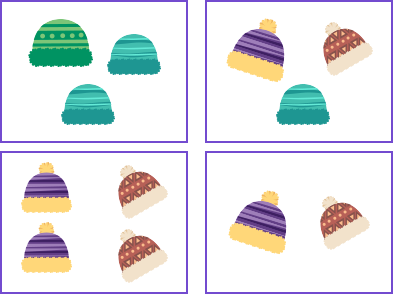Each group has 3 items, so it shows equal shares. All groups show a different number of items, so it does not show equal shares.

Equal shares of a whole:

We can also make equal shares of a whole by dividing it into equal parts.

A whole could be divided horizontally, vertically or diagonally to get equal shares. An example of cutting an object in equal shares is cutting a pizza or a cake into equal slices.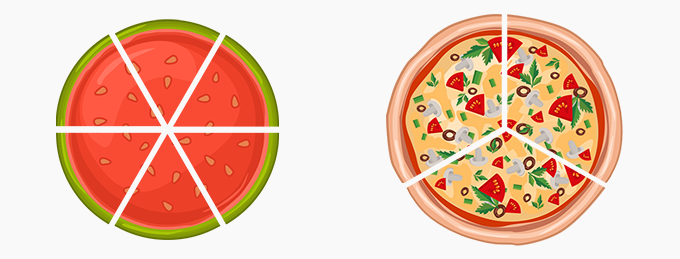Examples and non-examples of equal shares of a whole:

 Equal Shares Unequal Shares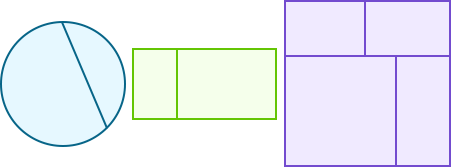Example: Distribute 1 cake equally among 4 friends.

As 1 cake needs to be divided among 4 people, we use 1 in the numerator and 4 in the denominator.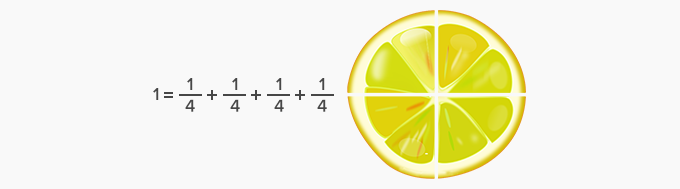This means that each friend gets a one-fourth share of the cake. It can also be said that 1 portion of the 4 equal portions is received by each friend.

 Fun Facts Equal shares when added together give us the whole. Equal shares can be used to divide either a single object or group of objects equally. The result of an equal share can sometimes be a fraction.
Won Numerous Awards & Honors